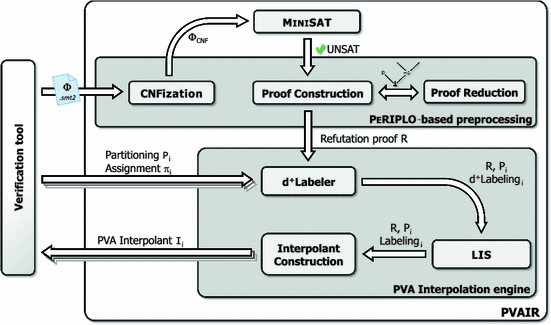# PVAIR

### Note: the functionality of PVAIR has been integrated to OpenSMT2 and is better maintained there!

Partial Variable Assignment InterpolatoR (PVAIR) is a tool exploiting variables assignments in the computation of interpolants. Partial variable assignments are used to specialize computed interpolants. Given a variable assignment, PVAIR is able to use the refutation proof of the input formula to compute interpolants for the unsatisfied parts of the formula. These interpolants can be considerably smaller than classical Craig Interpolants while still useful in many applications.

## Architecture

PVAIR is built on top of PeRIPLO. The input formula is first CNFized and passed to MiniSAT. In case of unsatisfiable formula, the refutation proof (in a DAG form) is constructed. Proof reduction techniques can be (optionally) applied to make the proof more concise. Then various variable assignments and partitionings of the input formula are used to compute Labellings for the given proof from which interpolants are finally derived.PVAIR (and some benchmarks) can be downloaded here. A tutorial describing basic usage can be found here.

### Related papers:

On Interpolants and Variable Assignments